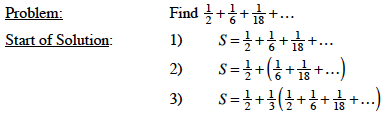### Home > CALC > Chapter 9 > Lesson 9.1.1 > Problem9-15

9-15.

Ying has a different method for summing an infinite series. Here are her steps:

1. Let $S =$ the sum.

2. Separate the first term from the rest of the series.

3. Factor the rest of the terms so that the remaining factor is $S$.

4. Substitute $S$ for the other factor.

5. Solve for $S$.

Apply Ying's method to find $S$ in the following problem. The first three steps are done for you. Copy them first, then complete the solution.$.075$